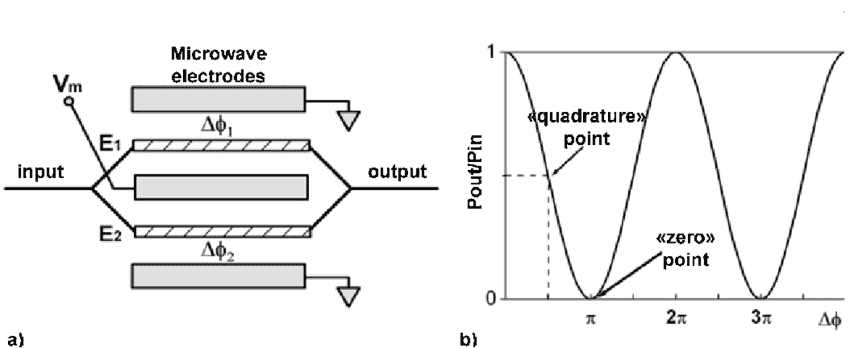# Controlling output light power by constructive/destructive interference

Mayan Fung
In optical communications, one of the modulation methods is to control the optical power (Simplest case, for example, bright = bit 1, dim = bit 0). I learned that we can achieve this by a Mach-Zehnder modulator (MZ modulator).

Simply speaking, the principle of MZ modulator is to split the input light into two, traveling in two waveguides. By applying a voltage across one of the two arms, we can alter the refractive index of it and thus inducing a phase difference between the two split lights. Then, when they are combined as one output light signal, if they constructively interfere, then the output power is maximum (bit 1). Otherwise, if they destructively interfere, then the output power is minimum (bit 0).

It sounds good till here. Yet, I am puzzled about energy conservation. Let's say we split the input light into two with the same amplitude. When they combined with destructive interference, there is no light. How come we have optical power input but no power output?The two point sources are combined so as to get cancellation in one direction.
There will be other directions in which the energy is summed.

Mayan Fung
The two point sources are combined so as to get cancellation in one direction.
There will be other directions in which the energy is summed.

But in the above schematic, seems that there is only one output direction. Can the light escape the wavelength?

But in the above schematic, seems that there is only one output direction.
The diagram is symbolic.
It is not shown how light is split into two channels.
It is not shown how light is recombined into one line.

•sophiecentaur